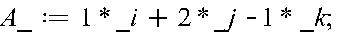Lab II Matrices

Do the following problems in classical dynamics by Marion and Thornton using Maple:

1-9 parts a-d

(Hints: use with(Physics[Vectors]): Setup(mathematicalnotation = true):

Vectors can be given defined asMagnitude is Norm; Dot product is just a period; Cross product is &x)

You may also use evalf and arccos functions.

1-10

(Hints: assume b,w, t to be real. You may use the following commands: VectorDiff(rad,t), V:= unapply(v,t)).

Instead of VectorDiff, diff may work better (seems the case for Maple 17)

1-11 - first part [Just demonstrate that the first equation that is written out are correct.]

(Hints, assume all componenets are real. This time use restart;

Setup(mathematicalnotation = true);

with(LinearAlgebra);

with(Physics[Vectors]);

One way to define a matrix is using M:= Matrix([[ A1 , A2 , A3 ],[ B1 , B2 , B3 ],[ C1 , C2 , C3 ]])

You may use the following commands: Determinant.

1-14 part a only

(Hints: You may use the commands: AB:= Multiply(A,B);).

IV Find the gradient of cos(x) + sin(z).

IV Let A = sin(x) i + cos(z) j + ex k. Find the divergence and curl of A.